## Light

Light

Light waves , radio waves and X-Rays, and gamma rays are all types of electromagntic waves.

The velocity of light in a vacuum (c) = 3.00 x 10 8 m/s.  The velocity of all magnetic waves are the same in a vacuum.

The brightness of a light source is called its luminous intensity (I )and the unit of brightness is the candela.  The candela is defined as the light emitted by a blackbody at the freezing temperature of platinum, (1773oC). The intensity of a light source is often called its candlepower.

The amount of light that falls on a given surface is called luminous flux (F) .   This has a unit of the lumen (lm).   One lumen is equal to the luminous flux which falls on each m2 of a sphere of 1m radius when a 1 cd isotropic light source is at the centre of the sphere.   The area of a sphere is 4 . p . r2

For a light source emitting light equally in all directions (isotropic) ..<./p>

Luminous Flux = 4 . p luminous intensity.
F = 4. p. I

Note :
The angle θ in radians is the length of the circle arc divided by the radius.
The solid angle Ω in steradians is the area of the surface of the sphere divided by the radius2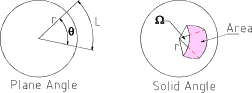The flux of a 1 cd source results in a luminous intensity of 1 lumen/ steradian.   The definition of liminous flux is ;-

Luminous Flux = F = I . Ω

The illumination (E) of a surface is the liminous flux per unit area that reaches the surface.   The unit of illumination is the lux (lx).

Illumination E (lx) = F (lm) /A (m2)

Reflection of Light

When a beam of light is reflected from a reflective plane surface the angle of reflection equals the angle of incidence.   The image has the same shape and size as the object but with left and right reversed. The image is perceived to be the same distance behind the mirror as the object is in front.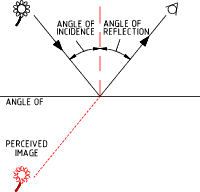Refraction of Light

The velocity of life is different in different mediums.  When a beam of light passes from one medium to another at an angle its direction changes.   The greater the differences in the velocity of light in the different mediums the greater is the angle.

The index of refraction of a transparent medium is the ratio between the velocity of light in free space (c) and the velocity of light in the medium (v)

Index of refraction = n = c/v

Snells Law = Sin i/Sin r = v1 / v2 = n2 / n1

 i = angle of incidence r = angle of refraction v1 = velocity of light in first medium v2 = velocity of light in second medium n1 = index of refraction of first medium n2 = index of refraction of second medium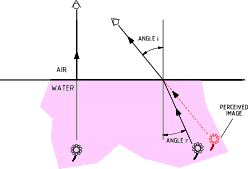The index of refraction varies with the frequency of light. White light comprises a number of primary colours all of which have a different frequency.   If light passes through a glass object with parellel sides the light will bend as it enters one side of the and bend back on leaving the object.   If it passes through an object which does not have parallel sides e.g a prism ,the light is effectively split into its primary colours.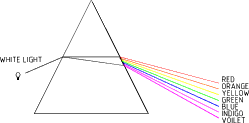If light passes from a medium of high refractive index to one of low refractive index e.g glass to air, there is an angle of incidence above which the angle of refraction is above 90o.    Above this angle (critical angle) the light is reflected back into the into the original medium.  This principle is used in the design of prisms used to bend light.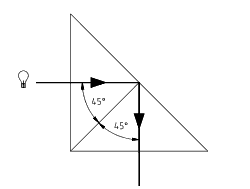## Sites Physics -Light

1. Optical Research Associates ....A compilation of useful Physics documents
2. GCSE Physics - Waves....Lots of very pretty and informative tutorials
3. Micro_Magnet ....Lens Java Tutorial.
4. U of Sheffied Light and Optics....Subject broken down to topics each including a comprehenisve presentation